SLOS270F March   2001  – August 2016

PRODUCTION DATA.

1. Features
2. Applications
3. Description
4. Revision History
5. Device Comparison Tables
6. Pin Configuration and Functions
7. Specifications
8. Detailed Description
1. 8.1 Overview
2. 8.2 Functional Block Diagram
3. 8.3 Feature Description
4. 8.4 Device Functional Modes
9. Application and Implementation
1. 9.1 Application Information
2. 9.2 Typical Application
10. 10Power Supply Recommendations
11. 11Layout
12. 12Device and Documentation Support
13. 13Mechanical, Packaging, and Orderable Information

• D|8
• DBV|5
• P|8
• D|8

## 7 Specifications

### 7.1 Absolute Maximum Ratings

over operating free-air temperature range (unless otherwise noted)(1)
MIN MAX UNIT
Voltage Supply voltage, VDD (2) 16.5 V
Differential input voltage, VID –VDD VDD
Input voltage, VI(2) –0.2 VDD + 0.2
Current Input current, IIN –10 10 mA
Output current, IO –100 100
Temperature Operating free-air temperature, TA: I-suffix –40 125 °C
Maximum junction temperature, TJ 150
Storage temperature, Tstg –65 150
(1) Stresses beyond those listed under Absolute Maximum Ratings may cause permanent damage to the device. These are stress ratings only, which do not imply functional operation of the device at these or any other conditions beyond those indicated under Recommended Operating Conditions. Exposure to absolute-maximum-rated conditions for extended periods may affect device reliability.
(2) All voltage values, except differential voltages, are with respect to GND.

### 7.2 Recommended Operating Conditions

over operating free-air temperature range (unless otherwise noted).
MIN MAX UNIT
Supply voltage, VDD Single supply 2.7 16 V
Split supply ±1.35 ±8
Common-mode input voltage, VCM 0 VDD V
Operating free-air temperature, TA I-suffix –40 125 °C
Turnon voltage (shutdown pin voltage level), V(ON), relative to GND pin voltage 2 V
Turnoff (shutdown pin voltage level), V(OFF), relative to GND pin voltage 0.8 V

### 7.3 Thermal Information: TLV2370

THERMAL METRIC(1) TLV2370 UNIT
DBV (SOT-23) D (SOIC) P (PDIP)
6 PINS 8 PINS 8 PINS
RθJA Junction-to-ambient thermal resistance 228.5 138.4 49.2 °C/W
RθJC(top) Junction-to-case (top) thermal resistance 99.1 89.5 39.4 °C/W
RθJB Junction-to-board thermal resistance 54.6 78.6 26.4 °C/W
ψJT Junction-to-top characterization parameter 7.7 29.9 15.4 °C/W
ψJB Junction-to-board characterization parameter 53.8 78.1 26.3 °C/W
RθJC(bot) Junction-to-case (bottom) thermal resistance n/a n/a n/a °C/W
(1) For more information about traditional and new thermal metrics, see the Semiconductor and IC Package Thermal Metrics application report.

### 7.4 Thermal Information: TLV2371

THERMAL METRIC(1) TLV2371 UNIT
DBV (SOT-23) D (SOIC) P (PDIP)
5 PINS 8 PINS 8 PINS
RθJA Junction-to-ambient thermal resistance 228.5 138.4 49.2 °C/W
RθJC(top) Junction-to-case (top) thermal resistance 99.1 89.5 39.4 °C/W
RθJB Junction-to-board thermal resistance 54.6 78.6 26.4 °C/W
ψJT Junction-to-top characterization parameter 7.7 29.9 15.4 °C/W
ψJB Junction-to-board characterization parameter 53.8 78.1 26.3 °C/W
RθJC(bot) Junction-to-case (bottom) thermal resistance n/a n/a n/a °C/W

### 7.5 Thermal Information: TLV2372

THERMAL METRIC(1) TLV2372 UNIT
D (SOIC) DGK (VSSOP) P (PDIP)
8 PINS 8 PINS 8 PINS
RθJA Junction-to-ambient thermal resistance 138.4 191.2 49.2 °C/W
RθJC(top) Junction-to-case (top) thermal resistance 89.5 61.9 39.4 °C/W
RθJB Junction-to-board thermal resistance 78.6 111.9 26.4 °C/W
ψJT Junction-to-top characterization parameter 29.9 5.1 15.4 °C/W
ψJB Junction-to-board characterization parameter 78.1 110.2 26.3 °C/W
RθJC(bot) Junction-to-case (bottom) thermal resistance n/a n/a n/a °C/W

### 7.6 Thermal Information: TLV2373

THERMAL METRIC(1) TLV2373 UNIT
DGS (VSSOP) D (SOIC) P (PDIP)
10 PINS 14 PINS 14 PINS
RθJA Junction-to-ambient thermal resistance 166.5 67 66.3 °C/W
RθJC(top) Junction-to-case (top) thermal resistance 41.8 24.1 20.5 °C/W
RθJB Junction-to-board thermal resistance 86.1 22.5 26.8 °C/W
ψJT Junction-to-top characterization parameter 1.5 2.2 2.1 °C/W
ψJB Junction-to-board characterization parameter 84.7 22.1 26.2 °C/W
RθJC(bot) Junction-to-case (bottom) thermal resistance n/a n/a n/a °C/W

### 7.7 Thermal Information: TLV2374

THERMAL METRIC(1) TLV2374 UNIT
D (SOIC) N (PDIP) PW (TSSOP)
14 PINS 14 PINS 14 PINS
RθJA Junction-to-ambient thermal resistance 67 66.3 121 °C/W
RθJC(top) Junction-to-case (top) thermal resistance 24.1 20.5 49.4 °C/W
RθJB Junction-to-board thermal resistance 22.5 26.8 62.8 °C/W
ψJT Junction-to-top characterization parameter 2.2 2.1 5.9 °C/W
ψJB Junction-to-board characterization parameter 22.1 26.2 62.2 °C/W
RθJC(bot) Junction-to-case (bottom) thermal resistance n/a n/a n/a °C/W

### 7.8 Thermal Information: TLV2375

THERMAL METRIC(1) TLV2375 UNIT
D (SOIC) N (PDIP) PW (TSSOP)
16 PINS 16 PINS 16 PINS
RθJA Junction-to-ambient thermal resistance 83 55.8 115.6 °C/W
RθJC(top) Junction-to-case (top) thermal resistance 44 43.1 50.5 °C/W
RθJB Junction-to-board thermal resistance 40.5 35.8 60.7 °C/W
ψJT Junction-to-top characterization parameter 11.5 27.9 7.4 °C/W
ψJB Junction-to-board characterization parameter 40.2 35.7 60.1 °C/W
RθJC(bot) Junction-to-case (bottom) thermal resistance n/a n/a n/a °C/W

### 7.9 Electrical Characteristics

at TA = 25°C, VDD = 2.7 V, 5 V, and 15 V (unless otherwise noted).
PARAMETER TEST CONDITIONS MIN TYP MAX UNIT
DC PERFORMANCE
VOS Input offset voltage At TA = 25°C, VIC = VDD/2, VO = VDD/2, RS = 50 Ω 2 4.5 mV
At TA = –40°C to +125°C, VIC = VDD/2, VO = VDD/2,
RS = 50 Ω
6 mV
dVOS/dT Offset voltage drift At TA = 25°C, VIC = VDD/2, VO = VDD/2, RS = 50 Ω 2 µV/°C
CMRR Common-mode rejection ratio VDD = 2.7 V,
RS = 50 Ω
VIC = 0 to VDD 50 68 dB
At TA = –40°C to +125°C,
VIC = 0 to VDD
49
VIC = 0 to VDD − 1.35 V 56 70
At TA = –40°C to +125°C,
VIC = 0 to VDD − 1.35 V
54
VDD = 5 V,
RS = 50 Ω
VIC = 0 to VDD 55 72
At TA = –40°C to +125°C,
VIC = 0 to VDD
54
VIC = 0 to VDD − 1.35 V 67 80
At TA = –40°C to +125°C,
VIC = 0 to VDD − 1.35 V
64
VDD = 15 V,
RS = 50 Ω
VIC = 0 to VDD 64 82
At TA = –40°C to +125°C,
VIC = 0 to VDD
63
VIC = 0 to VDD − 1.35 V 67 84
At TA = –40°C to +125°C,
VIC = 0 to VDD − 1.35 V
66
AVD Large-signal differential voltage amplification VDD = 2.7 V,
VO(PP) = VDD/2,
RL = 10 kΩ
98 106 dB
At TA = –40°C to +125°C 76
VDD = 5 V,
VO(PP) = VDD/2,
RL = 10 kΩ
100 110
At TA = –40°C to +125°C 86
VDD = 15 V,
VO(PP) = VDD/2,
RL = 10 kΩ
81 83
At TA = –40°C to +125°C 79
INPUT CHARACTERISTICS
IOS Input offset current VDD = 15 V,
VIC = VO = VDD/2
1 60 pA
At TA = 70°C 100
At TA = 125°C 1000
IB Input bias current VDD = 15 V,
VIC = VO = VDD/2
1 60 pA
At TA = 70°C 100
At TA = 125°C 1000
Differential input resistance 1000
Common-mode input capacitance f = 21 kHz 8 pF
OUTPUT CHARACTERISTICS
VOH High-level output voltage VDD = 2.7 V At TA = 25°C, VIC = VDD/2, IOH = −1 mA 2.55 2.58 V
At TA = –40°C to +125°C, VIC = VDD/2,
IOH = −1 mA
2.48
VDD = 5 V At TA = 25°C, VIC = VDD/2, IOH = −1 mA 4.9 4.93
At TA = –40°C to +125°C, VIC = VDD/2,
IOH = −1 mA
4.85
VDD = 15 V At TA = 25°C, VIC = VDD/2, IOH = −1 mA 14.92 14.96
At TA = –40°C to +125°C, VIC = VDD/2,
IOH = −1 mA
14.9
VDD = 2.7 V At TA = 25°C, VIC = VDD/2, IOH = −5 mA 1.9 2
At TA = –40°C to +125°C, VIC = VDD/2,
IOH = −5 mA
1.6
VDD = 5 V At TA = 25°C, VIC = VDD/2, IOH = −5 mA 4.6 4.68
At TA = –40°C to +125°C, VIC = VDD/2,
IOH = −5 mA
4.5
VDD = 15 V At TA = 25°C, VIC = VDD/2, IOH = −5 mA 14.7 14.8
At TA = –40°C to +125°C, VIC = VDD/2,
IOH = −5 mA
14.6
VOL Low-level output voltage VDD = 2.7 V At TA = 25°C, VIC = VDD/2, IOL = 1 mA 0.1 0.15
At TA = –40°C to +125°C, VIC = VDD/2,
IOL = 1 mA
0.22 V
VDD = 5 V At TA = 25°C, VIC = VDD/2, IOL = 1 mA 0.05 0.1
At TA = –40°C to +125°C, VIC = VDD/2,
IOL = 1 mA
0.15
VDD = 15 V At TA = 25°C, VIC = VDD/2, IOL = 1 mA 0.05 0.08
At TA = –40°C to +125°C, VIC = VDD/2,
IOL = 1 mA
0.1
VDD = 2.7 V At TA = 25°C, VIC = VDD/2, IOL = 5 mA 0.52 0.7
At TA = –40°C to +125°C, VIC = VDD/2,
IOL = 5 mA
1.1
VDD = 5 V At TA = 25°C, VIC = VDD/2, IOL = 5 mA 0.28 0.4
At TA = –40°C to +125°C, VIC = VDD/2,
IOL = 5 mA
0.5
VDD = 15 V At TA = 25°C, VIC = VDD/2, IOL = 5 mA 0.19 0.3
At TA = –40°C to +125°C, VIC = VDD/2,
IOL = 5 mA
0.35
IO Output current VDD = 2.7 V,
VO = 0.5 V from rail
Positive rail 4 mA
Negative rail 5
VDD = 5 V,
VO = 0.5 V from rail
Positive rail 7
Negative rail 8
VDD = 15 V,
VO = 0.5 V from rail
Positive rail 16
Negative rail 15
POWER SUPPLY
IDD Supply current (per channel) VDD = 2.7 V, VO = VDD/2 470 560 µA
VDD = 5 V, VO = VDD/2 550 660
VDD = 15 V,
VO = VDD/2
At TA = 25°C 750 900
At TA = –40°C to +125°C 1200
PSRR Power-supply rejection ratio (ΔVDD/ΔVIO) VDD = 2.7 V to 15 V,
VIC = VDD/2, no load
At TA = 25°C 70 80 dB
At TA = –40°C to +125°C 65
DYNAMIC PERFORMANCE
UGBW Unity gain bandwidth VDD = 2.7 V RL = 2 kΩ, CL = 10 pF 2.4 MHz
VDD = 5 V to 15 V RL = 2 kΩ, CL = 10 pF 3
SR Slew rate at unity gain VDD = 2.7 V At TA = 25°C, VO(PP) = VDD/2,
CL = 50 pF, RL = 10 kΩ
1.4 2 V/µs
At TA = –40°C to +125°C, VO(PP) = VDD/2,
CL = 50 pF, RL = 10 kΩ
1
VDD = 5 V At TA = 25°C, VO(PP) = VDD/2,
CL = 50 pF, RL = 10 kΩ
1.6 2.4
At TA = –40°C to +125°C, VO(PP) = VDD/2,
CL = 50 pF, RL = 10 kΩ
1.2
VDD = 15 V At TA = 25°C, VO(PP) = VDD/2,
CL = 50 pF, RL = 10 kΩ
1.9 2.1
At TA = –40°C to +125°C, VO(PP) = VDD/2,
CL = 50 pF, RL = 10 kΩ
1.4
φm Phase margin RL = 2 kΩ, CL = 100 pF 65 °
Gain margin RL = 2 kΩ, CL = 10 pF 18 dB
ts Settling time VDD = 2.7 V, V(STEP)PP = 1 V, AV = −1,
CL = 10 pF, RL = 2 kΩ, 0.1%
2.9 µs
VDD = 5 V, 15 V, V(STEP)PP = 1 V, AV = −1,
CL = 47 pF, RL = 2 kΩ, 0.1%
2
NOISE, DISTORTION PERFORMANCE
THD + N Total harmonic distortion plus noise VDD = 2.7 V VO(PP)= VDD/2 V, RL = 2 kΩ,
f = 10 kHz, AV = 1
0.02%
VO(PP)= VDD/2 V, RL = 2 kΩ,
f = 10 kHz, AV = 10
0.05%
VO(PP)= VDD/2 V, RL = 2 kΩ,
f = 10 kHz, AV = 100
0.18%
VDD = 5 V,
15 V
VO(PP)= VDD/2 V, RL = 2 kΩ,
f = 10 kHz, AV = 1
0.02%
VO(PP)= VDD/2 V, RL = 2 kΩ,
f = 10 kHz, AV = 10
0.09%
VO(PP)= VDD/2 V, RL = 2 kΩ,
f = 10 kHz, AV = 100
0.5%
Vn Equivalent input noise voltage f = 1 kHz 39 nV/√Hz
f = 10 kHz 35
In Equivalent input noise current f = 1 kHz 0.6 fA/√Hz
SHUTDOWN CHARACTERISTICS
IDD(SHDN) Supply current in shutdown mode (TLV2370, TLV2373, TLV2375) (per channel) VDD = 2.7 V, 5 V,
SHDN = 0 V
At TA = 25°C 25 30 µA
At TA = –40°C to +125°C 35
VDD = 15 V,
SHDN = 0 V
At TA = 25°C 40 45
At TA = –40°C to +125°C 50
t(on) Amplifier turnon time(1) RL = 2 kΩ 0.8 µs
t(off) Amplifier turnoff time(1) RL = 2 kΩ 1 µs
(1) Disable time and enable time are defined as the interval between application of the logic signal to the SHDN terminal and the point at which the supply current has reached one half of its final value.

### Table 3. Table of Graphs

FIGURE
VIO Input offset voltage vs Common-mode input voltage Figure 2, Figure 3, Figure 4
CMRR Common-mode rejection ratio vs Frequency Figure 5
Input bias and offset current vs Free-air temperature Figure 6
VOL Low-level output voltage vs Low-level output current Figure 7, Figure 9, Figure 11
VOH High-level output voltage vs High-level output current Figure 8, Figure 10, Figure 12
VO(PP) Peak-to-peak output voltage vs Frequency Figure 13
IDD Supply current vs Supply voltage Figure 14
PSRR Power supply rejection ratio vs Frequency Figure 15
AVD Differential voltage gain and phase vs Frequency Figure 16
Gain-bandwidth product vs Free-air temperature Figure 17
SR Slew rate vs Supply voltage Figure 18
vs Free-air temperature Figure 19
φm Phase margin vs Capacitive load Figure 20
Vn Equivalent input noise voltage vs Frequency Figure 21
Voltage-follower large-signal pulse response Figure 22, Figure 23
Voltage-follower small-signal pulse response Figure 24
Inverting large-signal response Figure 25, Figure 26
Inverting small-signal response Figure 27
Crosstalk vs Frequency Figure 28
Shutdown forward & reverse isolation vs Frequency Figure 29
IDD(SHDN) Shutdown supply current vs Supply voltage Figure 30
IDD(SHDN) Shutdown pin leakage current vs Shutdown pin voltage Figure 31
IDD(SHDN) Shutdown supply current, output voltage vs Time Figure 32, Figure 33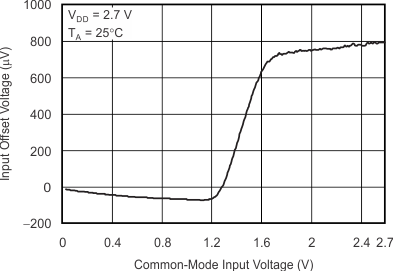Figure 2. Input Offset Voltage vs Common-Mode Input Voltage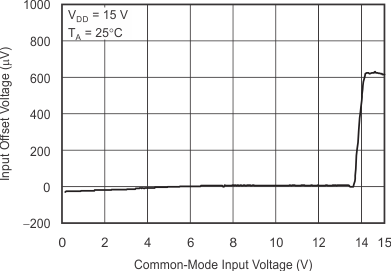Figure 4. Input Offset Voltage vs Common-Mode Input Voltage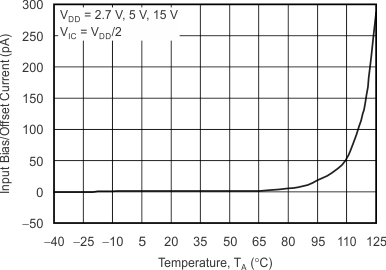Figure 6. Input Bias or Offset Current vs Free-Air Temperature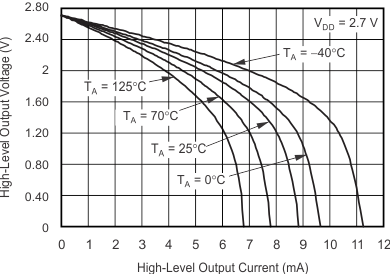Figure 8. High-Level Output Voltage vs High-Level Output Current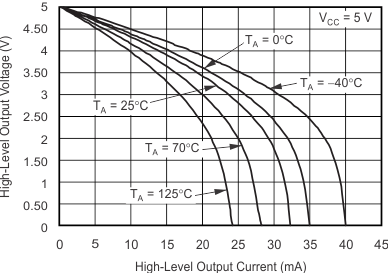Figure 10. High-Level Output Voltage vs High-Level Output Current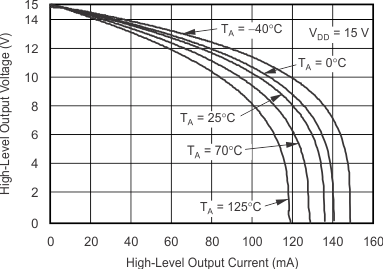Figure 12. High-Level Output Voltage vs High-Level Output Current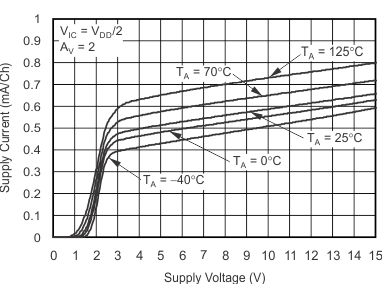Figure 14. Supply Current vs Supply Voltage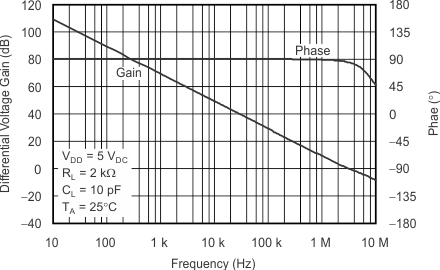Figure 16. Differential Voltage Gain and Phase vs Frequency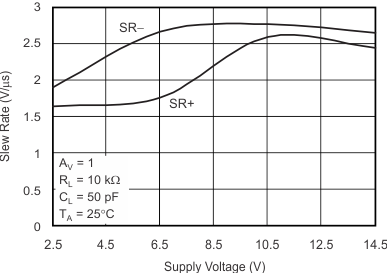Figure 18. Slew Rate vs Supply Voltage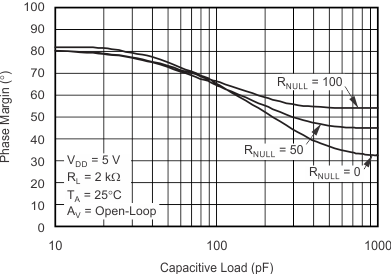Figure 20. Phase Margin vs Capacitive Load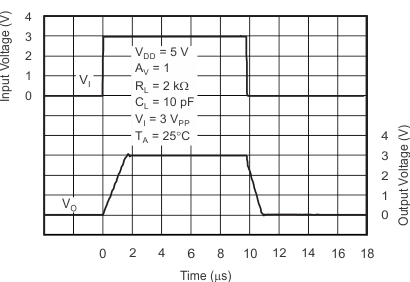Figure 22. Voltage-Follower Large-Signal Pulse Response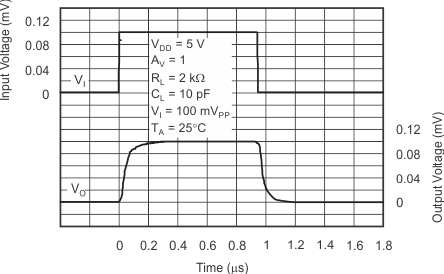Figure 24. Voltage-Follower Small-Signal Pulse Response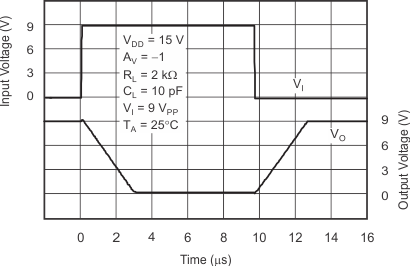Figure 26. Inverting Large-Signal Response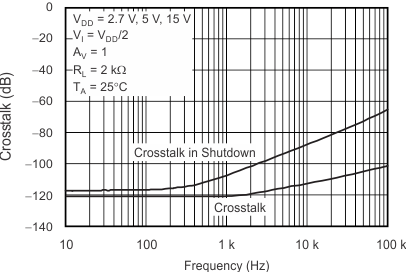Figure 28. Crosstalk vs Frequency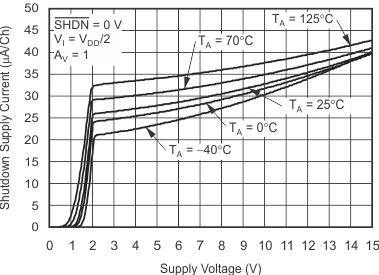Figure 30. Shutdown Supply Current vs Supply Voltage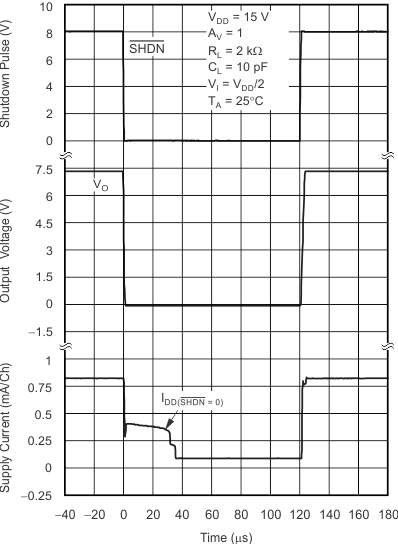Figure 32. Shutdown Supply Current and Output Voltage vs Time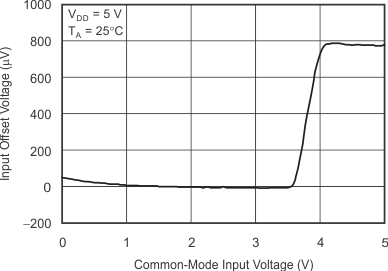Figure 3. Input Offset Voltage vs Common-Mode Input Voltage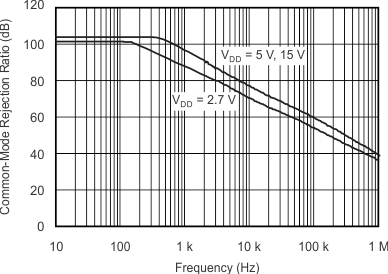Figure 5. Common-Mode Rejection Ratio vs FrequencyFigure 7. Low-Level Output Voltage vs Low-Level Output Current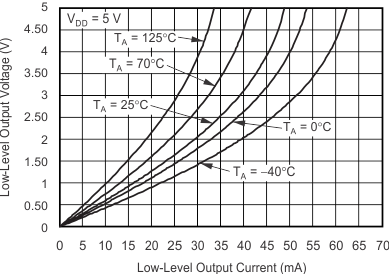Figure 9. Low-Level Output Voltage vs Low-Level Output Current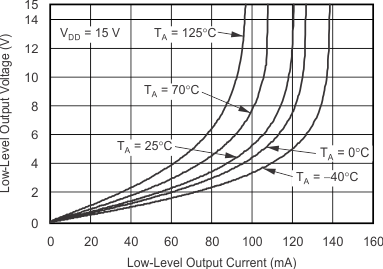Figure 11. Low-Level Output Voltage vs Low-Level Output Current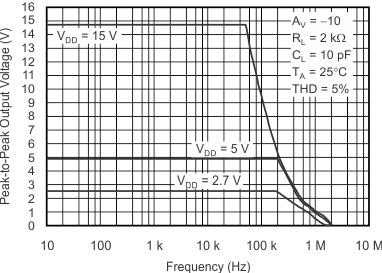Figure 13. Peak-to-Peak Output Voltage vs Frequency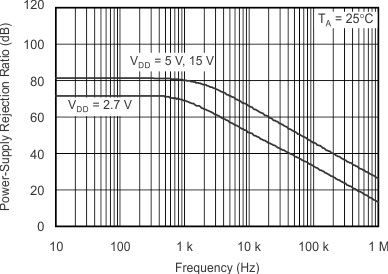Figure 15. Power Supply Rejection Ratio vs FrequencyFigure 17. Gain Bandwidth Product vs Free-Air TemperatureFigure 19. Slew Rate vs Free-Air Temperature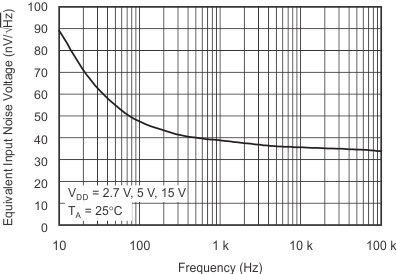Figure 21. Equivalent Input Noise Voltage vs Frequency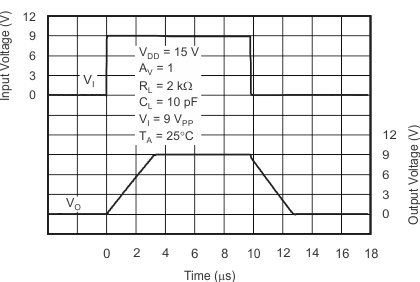Figure 23. Voltage-Follower Large-Signal Pulse Response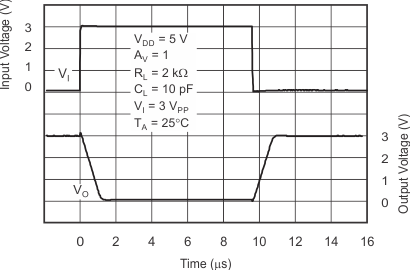Figure 25. Inverting Large-Signal Response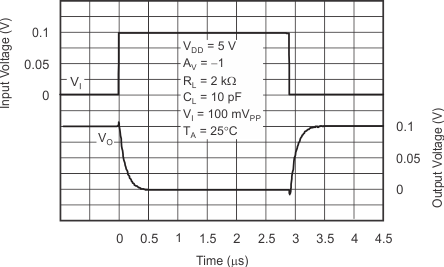Figure 27. Inverting Small-Signal Response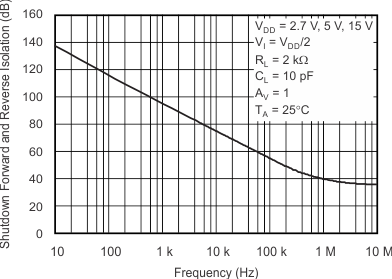Figure 29. Shutdown Forward and Reverse Isolation vs Frequency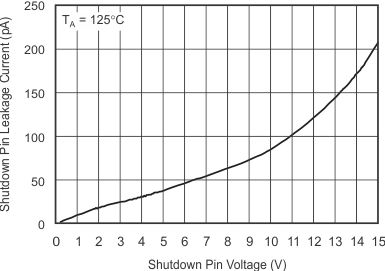Figure 31. Shutdown Pin Leakage Current vs Shutdown Pin Voltage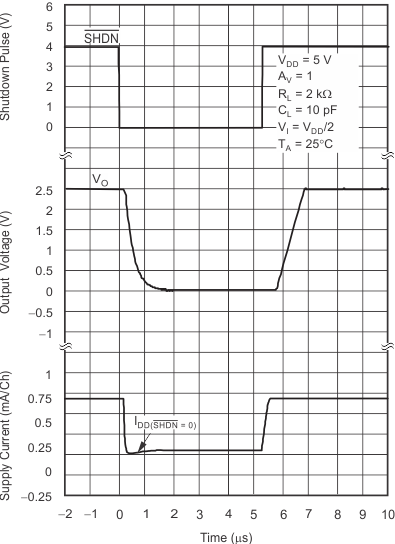Figure 33. Shutdown Supply Current/output Voltage vs Time